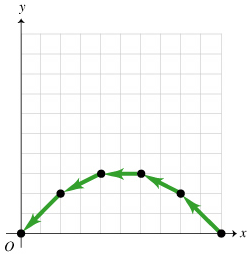# Problem: For the motion diagram given (Figure 1), sketch the shape of a possible graph for the y position versus time, y(t). Use the indicated coordinate system. One unit of time elapses between consecutive dots in the motion diagram.

###### FREE Expert Solution

Construct a possible graph for the y position versus time, y(t).

The change in y is not constant. Initially, y increases by 2 units, then 1 unit, then decreases by 1 unit and finally 2 units.

96% (44 ratings)###### Problem Details

For the motion diagram given (Figure 1), sketch the shape of a possible graph for the y position versus time, y(t). Use the indicated coordinate system. One unit of time elapses between consecutive dots in the motion diagram.Frequently Asked Questions

What scientific concept do you need to know in order to solve this problem?

Our tutors have indicated that to solve this problem you will need to apply the Symmetrical Launch concept. You can view video lessons to learn Symmetrical Launch. Or if you need more Symmetrical Launch practice, you can also practice Symmetrical Launch practice problems.

What professor is this problem relevant for?

Based on our data, we think this problem is relevant for Professor Thurston's class at Oklahoma City Community College.